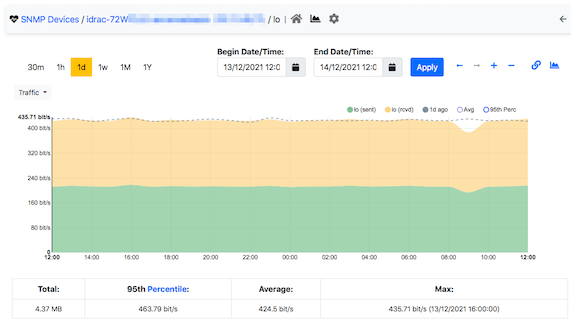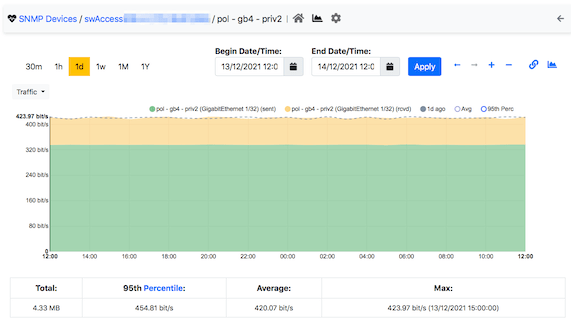# A Gentle Introduction To Timeseries Similarity in nDPI (and ntopng)

### Introduction

Let’s start from the end. In your organisation you probably have thousand of timeseries of various nature: SNMP interfaces, hosts traffic, protocols etc. You would like to know what timeseries are similar as this is necessary for addressing many different questions:

• Host A and host B are two different hosts that have nothing in common but have the same traffic behaviour.
• Host C is under attack: who else is also under attack?
• SNMP interface X and interface Y are load balancing/sharing the same traffic: is their timeseries alike or not? Namely is load balancing working as it should?
• Is host Z behaving differently with respect to last week?

In essence we want to spot timeseries that are similar (ignore for a second the case where they are exactly the same, such as two timeseries with constant traffic) such as those shown below.In the latest ntopng we have introduced (currently limited to SNMP interfaces but soon it will be extended to other components, a new function that allows you to show what SNMP interfaces have a similar timeseries and mark them with a similarity score (the higher the more similar they are). Using this feature you can find in your list of devices what ports behave the same way and thus find similarities (or unexpected non-similarities) in a matter of clicks. All this is implemented on top of nDPI that features all the necessary tools for implementing all this as described in the next section without complex/heavy machine learning techniques that would prevent ntop tools from running on all devices.### Detecting Timeseries Similarity in nDPI

nDPI is often known only for detecting application protocols. However it also implements various methods and algorithms for analyzing data. Today we’ll talk about timeseries similarity detection in nDPI. A timeseries is a time-ordered list of data points. Detecting similarity in timeseries is a complicated task (see this document for reading more about this subject) but at the same time we need to find an easy solution that allows it to be implemented at low cost. nDPI implements data binning techniques that can make this task easy. In networking, timeseries are all created at the same time (e.g. by ntopng) so there is no need to shift the time series by aligning them using algorithms such as dynamic time warping, this unless you are comparing timeseries created at different timezones that is not our case. Two timeseries can be compared as follows: for every point in the series, we compute the sum of the square of the two timeseries difference as shown in the table below.

 Series A Series B Difference Difference^2 12 11 1 1 34 32 2 4 23 22 1 1 43 45 -2 4 23 23 0 0 Total 10

nDPI implements bins and thus once a timeseries has been converted into a bin, it can be easily compared. Two timeseries are identical if the total is zero: the larger is the total the more different are the timeseries. In many networks, you need to compare thousand of series, hence a dummy 1:1 timeseries comparison would be inefficient having a squared complexity. However, this algorithm can be simplified by implementing and early discard of possible timeseries combinations that wouldn’t match. This is possible as follows:

• For each bin (read it as timeseries) use nDPI to compute the average and standard deviation.
• Represent each timeseries as a circle whose center is placed on the X axis at a distance from origin of its average value, and a radius of its standard deviation.
• Two timeseries are definitively not similar if the two circles do not intersect (the opposite is not true either).Using this technique it is possible to avoid comparisons that would not result in similarity and focus only on those that can likely match. To make this long story short and see how all this work in practice, we have written a simple tool (this is the complete source code) that using timeseries written in RRD format by ntopng can find similarities. To give you an idea of how long this process takes, on a old dual Intel Core i3 can read 2500 host timeseries and compare them in less than a second. This is the same algorithm used by ntopng to compare SNMP interfaces traffic listed earlier in this article and that is also as fast as this simple similarity tool.

Enjoy !